This page examines an argument I have seen presented as a technical ‘justification’ for claiming that bi-wiring is different to conventional loudspeaker wiring. Specifically because the resistance of conventional wiring is claimed to produce a form of distortion. An example of this argument appears in a web forum thread that starts at:

The owners of the forum have given me permission to quote the thread and use it as a basis for the following.

The Claim
The central points of the argument are summarised in posting 99 of the above thread so I will quote them below. You may wish to visit the forum and examine the thread to put the following in context, but the quote should suffice for the purpose of what follows:

 The currents and the resistance are normalized, so the peak woof and tweet currents are 1, and the resistance of the wire is also normalized to 1. The woof current is the blue line, the diss is (Asquared) I2R, so as you can see, it peaks at 1, and is always positive (real dissipation cannot be negative). It is your basic sine squared waveform, a direct result of the woof current flowing through a wire by itself. Note that for an ideal load, this is also exactly the same dissipation time profile scaled differently. The tweet current is the magenta line, called Bsquared. again, I squared R... With biwiring, the total wire dissipation is of course, the sum of the two, A squared plus B squared, and the load dissipation at the speakers is an exact scale of the wire dissipation. The yellow line (asq plus b sq) is the summation of the wire loss in biwiring. Now, consider both signals travelling in one wire, such as monowiring.. The equation is P = I2R, with I = A + B (A+B)2 = A2 + B2 + 2AB. So, subbing A + B for I creates 3 parts of the power dissipation, the third and most interesting component being the 2AB part.. I put that on the graph, that is the brown (I think) color. Note two very important things about the 2AB component... 1. It goes NEGATIVE!! At first blush, that seems impossible..However, look at it's value compared to the yellow line which is the A2 + B2 part....note that if you sum them, they NEVER go negative. In other words, the 2AB component is a modulation of the expected dissipation. So, the monowire dissipation never goes negative.. 2. It is a ZERO INTEGRAL power waveform...in other words, what is below zero is exactly the same area as that above zero. Since FFT algorithms cannot spot a zero integral power waveform, it isn't seen. Think of the instant in time when the woof has 1 ampere positive and the tweet has one ampere negative...at that instant, a monowire sees zero current, therefore zero power loss within the wire... But, a biwire setup has one ampere in the bass wire with it's dissipation, the tweet wire has negative one ampere and the exact same loss as the bass wire... The result? In a monowire setup, the current of one signal will modulate the losses that are caused by the other..when I see loop resistance recommendations at the 5% level, I cringe..

The conclusion of the above argument is that the current for one signal component “modulates” that for the others, and hence creates a form of ‘intermodulation distortion’ (as is claimed in other postings in the web forum thread). But does the above ‘explanation’ make any sense? Does it accurately describe the physics of the system being described? If it does, then bi-wiring may sound different to conventional wiring by avoiding the claimed distortion.

Simple System – Simple Analysis.
The key question here is, “Does the claimed form of ‘intermodulation distortion’ arise in the conventionally wired system as described?” If it doesn’t actually occur, we need not bother with a similar analysis of bi-wiring because the reason given for needing it is void. As in the thread which prompted these pages I will use a simple model which considers the cable as being a series resistance, and the loudspeaker as being a resistive load. This arrangement is shown in the circuit schematic below.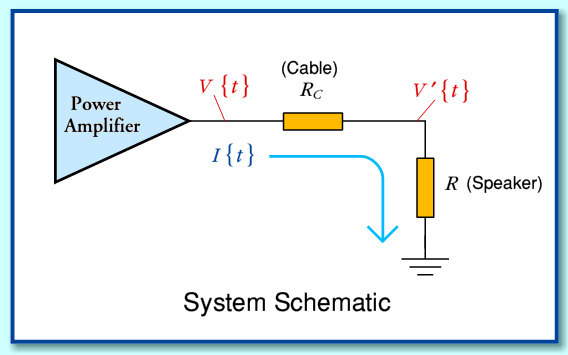Here we can use the symbols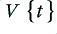output voltage from amplifier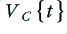voltage difference between ends of cable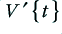voltage asserted at speaker (load) terminals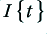current flowing through cable and load.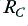cable series resistance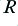speaker (load) resistance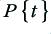Power output from amplifier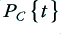Power dissipated in cable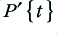Power dissipated in speaker (load)

Where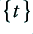indicates the value is a time-dependent variable.

It is worth making three basic points before continuing.
• Firstly, that it is the signal patternwhich defines the sound pressure pattern which we wish to emerge from the speaker.
• Secondly, that in the system considered, the current will always be proportional to this voltage once values for the resistance have been chosen.
• Thirdly, that the current levels in the cable and load are always identical as they are in series.

We can now compare two situations. One where we have an ‘ideal’ cable – i.e. a cable of zero series resistance. (e.g. one of zero length.) The other where we have a ‘real’ cable of non-zero resistance. For the sake of those who have an aversion to readings pages of algebra I have put the details in an appendix at the end of these pages and will now move to the actual comparison of the ‘ideal’ and ‘real’ cases. The results are summarised in the table below.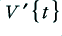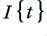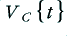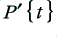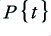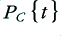‘ideal’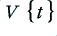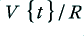0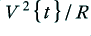0 ‘real’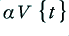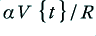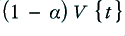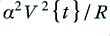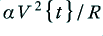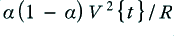Note that as explained in the appendix, the above expressions have been simplified for the sake of comparison by using the factor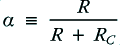Comparing the values we can see that the effect of the cable having a non-zero resistance in this simple resistive system is to scale the powers and voltages by a amounts which are fixed once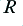andhave been set. As you would expect,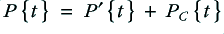and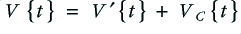throughout, and so power is conserved and the voltages add correctly.

To an engineer or physical scientist the above all confirms that this is a linear system, and that the effect of the cable resistance is simply to act as an attenuator or volume control which reduced the speaker voltage by a factor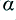. However to make this clearer we can now show the results in a a graphical form.

It’s all a Plot.
The situation described in the claim is one where a signal consists of two frequency components which are referred to as ‘A’ and ‘B’. Lets use this as an example and plot the results we get. For the sake of example, consider the case where the signal from the amplifier consists of two sinusoids, each of amplitude 10 Volts at the amplifier output, with frequencies of 200Hz and 250Hz. The loudspeaker load has a resistance of 10 Ohms, and the cable has a series resistance of 1 Ohm. (I have deliberately chosen a large cable resistance to make the effects clearer on the graphs.)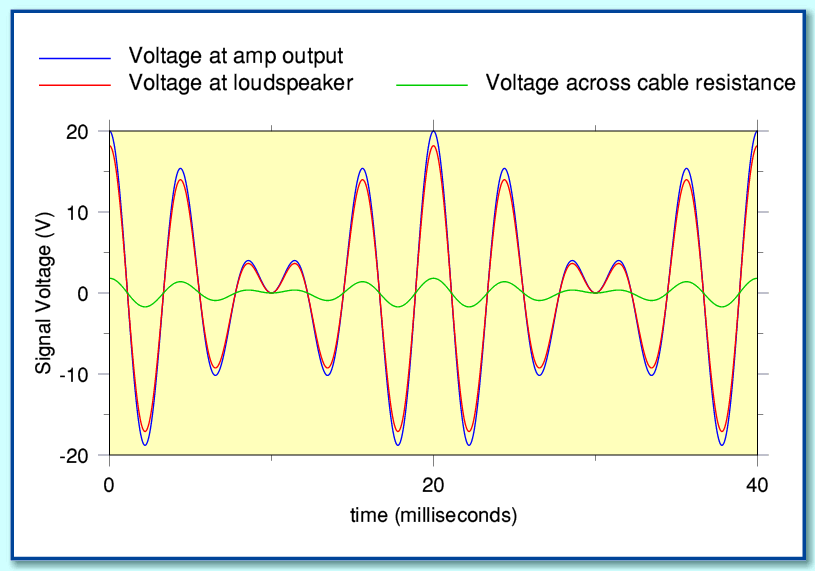The above shows the signal voltage patterns at the amplifier (blue), and loudspeaker (red). The voltage difference between the ends of the cable resistance is also shown (green). The graphs show the same shape for all three patterns. The only difference being that the voltage at the speaker terminals is reduced in size by the cable and speaker acting as a potential divider. (i.e. as shown in the equations above, the signal voltage is scaled by.) The waveforms have the same shapes, and the same spectral components except for this overall change in size. By Ohm’s Law, the current must also adopt the same pattern. The above shows no sign of any ‘intermodulation distortion’ effects being caused by the cable resistance.

In another posting (166) in the web forum thread the following claim is made:

 ..that 2AB product I detailed, is the product of two frequencies, so there are frequencies produced that are not the fundamentals...

However as can be seen from the above, no such frequencies “not in the fundamentals” appear in the voltage waveforms. Let’s now also look at the situation with the powers as these are also discussed in the thread. The plots below show the time-variations of the power levels in our example.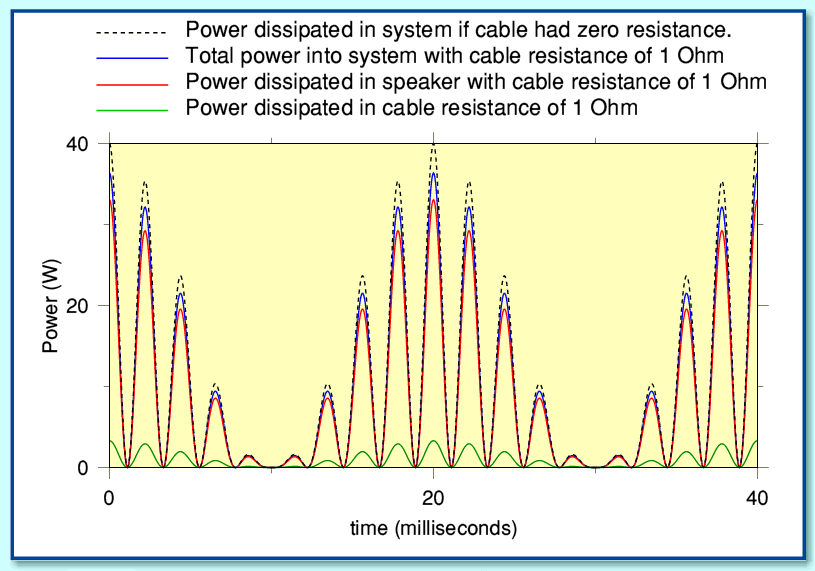The blue line shows the total system (loudspeaker plus cable) power level provided by the amplifier when the cable is as described above. The red line shows the power delivered to the speaker via the chosen cable. The green line shows the dissipation in the cable. For comparison, the broken black line shows the power dissipated if the cable were of zero resistance (e.g. of zero length) – i.e. the ‘ideal’ case. Note that when the cable has zero resistance this total power is identical to the speaker power since none can be lost in the cable!

As with the voltages, it can be seen that all these patterns are simply scaled versions of rhe same shape. No components or details are altered by the use of the cable apart from this linear scaling. All that happens is that the level of the signal at the speaker is attenuated by the cable having a non-zero resistance. The cable’s effect is indistinguishable from a slight adjustment of a volume control to reduce the overall sound level by a slight amount.

Given the above it should be clear that the nothing like ‘intermodulation distortion’ as described in the claim occurs in the arrangement being described. No extra frequency components appear as a result of the cable resistance dissipating power. As a consequence ‘bi-wiring’ need not be employed to deal with what the claim asserts. A problem that does not exist does not require a ‘solution’!

Indeed, if the claimed problem did exist, then bi-wiring would not be a satisfactory solution for the following reasons:

Bi-wiring may allow us to divide the spectrum into two bands. But that does not mean that we then only ever have one sinusoidal component in each band. In reality, music and speech will have a complex and varying spectrum. Hence we can generally expect both the LF and HF units of a speaker (and as a result any bi-wires to them) to have to carry multiple frequencies at the same time. Thus if the problem existed, it would still occur with bi-wired arrangements. To avoid this we’d have to have an undefined multiplicity of wires and speaker units, each dedicated to a single frequency. The same problem would also arise with all conventional volume controls, etc.

The above is all consistent with what is in the textbooks, and the results of measurements. It may or may not be the case that bi-wiring has some other effect. Indeed, as discussed elsewhere it can be shown to have an effect on the frequency response. However that is a linear effect, not a nonlinear distortion. Hence the above does not show that bi-wiring cannot possibly make any difference. It does show, however, that the claim simply does not stand up as an ‘reason’ for bi-wiring making a difference. The distortion mechanism as presented in the claim does not exist.

So what’s wrong with the claim?
To see why the claim is wrong, let’s examine it again. For convenience I’ve re-quoted some of the key parts below.

 [snip] The woof current is the blue line, the diss is (Asquared) I2R, so as you can see, it peaks at 1, and is always positive (real dissipation cannot be negative). [snip] The tweet current is the magenta line, called Bsquared. again, I squared R... With biwiring, the total wire dissipation is of course, the sum of the two, A squared plus B squared, and the load dissipation at the speakers is an exact scale of the wire dissipation. The yellow line (asq plus b sq) is the summation of the wire loss in biwiring. Now, consider both signals travelling in one wire, such as monowiring.. The equation is P = I2R, with I = A + B (A+B)2 = A2 + B2 + 2AB. So, subbing A + B for I creates 3 parts of the power dissipation, the third and most interesting component being the 2AB part.. [snip]

The claim describes the signal as two components, ‘A’ and ‘B’ but then does not really go on to give a detailed definition of these, beyond indicating they have different frequencies.

Keeping consistent with the meanings of the terms I used earlier, let’s now write out a specific example for the waveform, on the basis that it contains two sinusoids of different frequencies as used for the plots I presented earlier. This would have a form like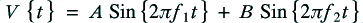Where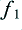and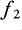are the chosen pair of signal component frequencies.

In a ‘real’ case (i.e. one whereis non-zero) this means the current will be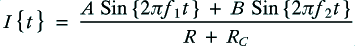This same current level will be present in both the cable and the load as they are in series. The power levels dissipated in the cable and the load will therefore both be equal to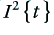multiplied by the appropriate resistance value in each case. This means each power vary with time with a pattern such that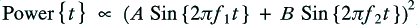i.e.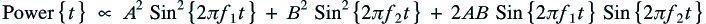As pointed out in the claim, this does contain an ‘AB’ term which isn’t simply a sinusoid at one of the original frequencies present in. However also note the sin-squared terms. If we expand the above using standard trig identities we get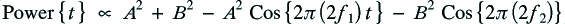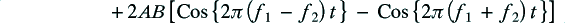This expressions shows the frequencies of the fluctuations present in the instantaneous power levels. Note that it does not contain any components at the frequenciesorat all! The frequency components in the power expression are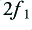,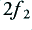,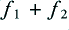, and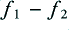. Indeed, even if we were to set one amplitude (e.g. ‘B’) to zero, we’d still find that the power fluctuations are at a different frequency to the voltage and current fluctuations. Allow me to emphasise the following point:

The above is standard behaviour for the square-law relationship between power and current or voltage.

All that is happening is that we are confirming that the power fluctuations have a non-linear relationship (squaring) with the voltage or current. The presence of the ‘AB’ term is simply a consequence of this. It tells us nothing about the ‘effect’ of a cable resistance since the same is true even if we have a cable of zero length and zero resistance. It is therefore a misdirection or muddle to assume that the ‘AB’ terms represents some sort of ‘intermodulation distortion’. To assume this, as in the claim, is simply to misunderstand the physics involved, and the relationship between current or voltage and power.

In reality, the loudspeaker is nominally designed to respond to the voltage pattern applied to its terminals. It uses some of the power delivered to do this, but the response should be proportional to the voltage pattern. Given this, and the details explained above, it is not a ‘problem’ that the power variations contain fluctuation frequencies which differ from those in the voltage pattern. It is no more than a normal consequence of the power varying with the square of the voltage. As shown both by the equations, and by the plots, the voltage (and current) patterns are scaled in amplitude by the resistance, but no ‘new’ frequencies are created by the presence of cable resistance.

Conclusions
To someone innocently reading the claims made in the web forum thread the effect is like being presented with a conjuring act. The result tends to mislead by having attention focus in the wrong direction. In this case this process may be summarised as:
• The claim muddles time variations in the power level with time variations in the voltage and current.
• It confuses the effect of a square-law relationship between current or voltage and power with a claimed effect of the presence of cable resistance.
• It directs attention onto the power dissipation in the cable, and away from considering the signal voltage, current, and power patterns at the speaker.
• Focussing on the cable, it does not notice that the voltage, current, and power patterns at the speaker and amplifier output have the same shapes with or without the cable resistance.

The claim is therefore based on a misunderstanding of – and hence a misrepresentation of – the relevant physics, and how details of the signal carry the required information. The reality, as shown by the above equations and graphs and – more importantly – by measurements, is that no form of ‘intermodulation distortion’ arises in the situation described.

As can be seen in the quoted postings, the web forum thread also includes various comments about “FFT” based measurements, etc. Unfortunately, the comments are generally either irrelevant, inappropriate, or plain incorrect. They stem from the claim having to explain away the simple fact that measurement results conflict with the claim. This has nothing to do with any ‘limits’ of Fourier Transformation or other methods - unless you regard the inability to detect something that not actually exist as a ‘limitation’!

Finally, it is perhaps pointing out that there is a physical mechanism by which the cable resistance value may vary dynamically in use, and that this could produce nonlinear alterations of the signal. This stems from the temperature dependence of the resistivity of the cable, and the heating effect of the dissipated power. However it seems unlikely that this is what anyone presenting the claim I have analysed has in mind because the dynamics and behaviour of this would be quite different to what is described in the postings I have quoted. In addition, with any reasonable choice of cable, such thermal effects would be tiny, and orders of magnitude smaller than similar effects inside the loudspeaker itself!

Appendix - The Maths!
Since the ‘ideal’ cable has zero resistance it immediately follows that in this case we can expect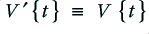, and that all the power provided by the amplifier will be dissipated or radiated by the loudspeaker load – i.e. that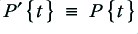in this ‘ideal’ case. The current in the loudspeaker will be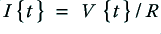, and the power will be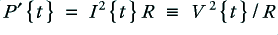.

This situation should then represent the ideal one where the speaker is driven exactly as we intend. We can therefore use what happens in this ‘ideal’ case to indicate the waveform shapes, etc, we wish to present to the speaker.

In the ‘real’ case the non-zero resistance has two effects.
• Firstly, the current will be reduced because we have increased the resistance presented to the amplifier fromto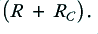• Secondly, this reduced current will produce a smaller voltage as it passes though the load (speaker) resistance. (Ohm’s Law.)

In the ‘real’ case we therefore find that the speaker load will experience an applied voltage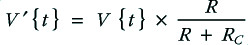and the current through it will be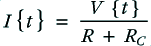Hence the power delivered into the speaker load will be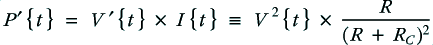and the total power supplied (i.e. including that dissipated in the cable) will be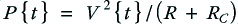The current in the cable is identical to that in the load. Hence the voltage drop between the amplifier and load ends of the cable will be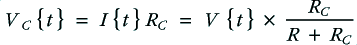The power dissipated in the cable will be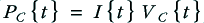To clarify the situation we can define the factorwhose value is set once we have chosen the cable and speaker resistances. i.e.is a fixed value for a given system. This factor can be used to simplify the expressions and make them more easily comparable. For example, we can now write that for the ‘real’ case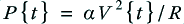and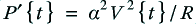and that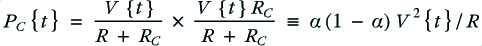The values can then be compared and plotted as explained in the main text.

Back to main Analog and Audio page.